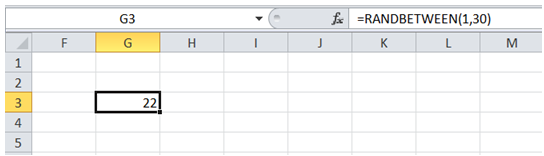## Random number between 2 limits in Excel

For example, I am setting up a quiz game for kids. I have 30 questions. I would like to choose one random number between 1 and 30 to start the quiz.

To do it in Excel, here is the answer:

a) Enter the formula =RANDBETWEEN(1,30) in an empty cell.

Note: Every time the workbook is recalculated, the cell containing the RANDBETWEEN formula is also recalculated. Hence the value in this cell is not STATIC.